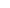Formule

Tesla Coil
formule that I have found useful

Ohm’s Law
 V = I * Z P = I * V

V = volts
I = current in amps
Z = impedance or resistance in ohms
P = power in watts

Non Resonant Transformer Input and Output

EP*IP = ES*IS

EP = primary voltage
IP = primary current in amps
ES = secondary voltage
IS = secondary current in amps

Capacitive Reactance

XC  =  1 / ( 2 ** F * C )

XC = capacitive reactance in ohms
F = frequency in hertz

Inductive Reactance

X =  2 ** F * L

XL = inductive reactance in ohms
F = frequency in hertz
L = inductance in henrys

Resonant Circuit Formula

F = 1/( 2 ** ((L * C))

F = frequency in hertz
L = inductance in henrys

Spiral Coil Inductance

 L = ( N*R )2 / ( 8*R + 11*W ) L = inductance of coil in microhenrys (�H) R = average radius of the coil in inches N = number of turns W = width of the coil in inches

Helical Coil Inductance

 L = ( N*R )2 / ( 9*R + 10*H ) L = inductance of coil in microhenrys (�H) N = number of turns R = radius of coil in inches H = height of coil in inches

Inverse Conical Coil Inductance

 L1 = ( N*R )2 / ( 9*R + 10*H ) L2 = ( N*R )2 / ( 8*R + 11*W ) L =( (L1* sin(x))2 + (L2* cos(x)) 2 ) L = inductance of coil in microhenrys (�H) L1 = helix factor L2 = spiral factor N = number of turns R = average radius of coil in inches H = effective height of the coil in inches W = effective width of the coil in inches X = rise angle of the coil in degrees

Medhurst

C = 0.29 * L + 0.41 * R + 1.94 *(R3 / L )

C = self capacitance in picofarads
R = radius of secondary coil in inches
L = length of secondary coil in inches

Toroid Capacitance

C = 1.4 * ( 1.2781 – (D2 / D1) )(* D2 * (D1 – D2) )

D1 = outside diameter of toroid in inches
D2 = diameter of cross section of toroid in inches

C = (25.4*R) / 9

Plate Capacitors

C =( .224 * K * A ( N -1) ) / (1000000 * D )

K = dielectric constant
A = area of each plate in square inches
N = number of plates
D = distance between plates in inches (thickness of dielectric)

Energy Stored in a Capacitor

J = 0.5 * V2 * C

J = joules of energy stored
V = peak charge voltage
I = peak current

Energy for and Inductor

J = 0.5 * I2 * L

J = joules of energy stored
V = peak charge voltage
I = peak current
L = inductance in henries

Inductance of a circular loop of wire (Wheeler)

Lo = Inductance of  loop

Lo = m0* a * (ln((8 * a) /R) -2)
Inductance of two parallel wires (Wheeler)

l = Length of wires
d= spacing of wires
Lo = Inductance of  wires.

Lo = (m0* l / d) * (ln(d / R) -1)
Inductance of two parallel inductors

Lt = Total Inductance
Lx = Inductance
M = mutual inductance between inductors

1/Lt = (1 / (L1* [+/-]M)) + (1 / (L2* [+/-]M))
Inductance of two series inductors

Lt = Total Inductance
Lx = Inductance
M = mutual inductance between inductors

Lt = (L1 + L2) + [+/-]2M)
Magnetic field around a single conductor

I=Current in wire
B=Magnetic Field Strength

B=(m0* I) / (2 ** R)

Resonant Transformer Voltage Multiplication from Inductance

Vs= Voltage on Secondary
Vp= Voltage on Primary
Ls= Inductance of Secondary
Lp= Inductance of Primary

Vs= Vp(Ls / Lp)

Resonant Transformer Voltage Multiplication from Capacitance

Vs= Voltage on Secondary
Vp= Voltage on Primary
Cs= Inductance of Secondary
Cp= Inductance of Primary

Vs= Vp(Cp / Cs)

Current Transformer Turns to Current ratio.

Ip= Primary Current
Is= Secondary Current
Np= Turns on Primary
Ns= Turns on Secondary

Ip/ Is= Ns/ Np